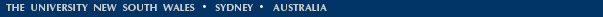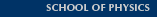# Aliasing and 'strange spectral components'

 We were sent this question: 'Lately I've been experimenting with old digital samplers in my music (Casio SK-1s, Yamaha VSSs, etc. They're cheap, fun, and not too hard to come by if you frequent the right thrift stores.) Part of the appeal is that these keyboards seem to add strange, ghostly harmonics when you play back samples through them. I read that these harmonics might be a result of a phenomenon called aliasing or folding. Could you explain what that is and how it affects digital audio?'. John Smith answers: Aliasing can occur when we measure a signal at a number of discrete instants in time. This is called the sampling frequency, and for audio measurements it is determined by the recording electronics. If we start by considering a simple sine wave as the input signal, we can only describe it accurately if we make at least two measurements every cycle. This means that any signal we sample with a frequency that is greater than one half of the sampling frequency, will not be well defined. This upper frequency limit is known as the Nyquist frequency. So, for an example, let’s assume that we make measurements with a sampling frequency of 8 kHz. The Nyquist frequency will thus be 8 /2 = 4 kHz. If we sample a pure sine wave of frequency 3 kHz, this is less than the Nyquist frequency of 4 kHz and it will be reproduced accurately when played back. However, if we sample a 5 kHz sine wave it turns out that the measurements will be exactly the same as when we measured the 3 kHz sine wave – this 3 kHz signal is an ‘alias’ of the original 5 kHz signal. So, if we now play back our recorded 5 kHz signal we get a 3 kHz signal rather than the original 5 kHz input signal; it has been ‘folded back’ at the Nyquist frequency. Indeed, for a sine wave with a frequency that lies between the Nyquist frequency and the sampling frequency, the alias will be a sine wave with a frequency that is as far below the Nyquist frequency as the input signal was above it. Another way to think of this is that the alias will be at a frequency given by the sampling frequency (i.e. twice the Nyquist frequency) minus the input frequency. So far we have considered only a simple sine wave input as the input, however most input msical signals will be more complicated and contain harmonics. Now the consequences of aliasing can become much more interesting. For most sounds with a fundamental frequency of frequency f, these harmonics will be in the ratio f, 2f, 3f, 4f, etc; the usual harmonic series. However, once aliasing occurs, the higher harmonics need no longer be in the harmonic series. Let’s consider an input signal with fundamental frequency of 1.5 kHz that contains 4 harmonics; the frequencies will be 1.5, 3, 4.5, and 6 kHz. The frequency ratios will be 1:2:3:4 and it will sound like a normal instrument. If it is sampled at 8 kHz, the component at 4.5 kHz will have an alias at 3.5 kHz (i.e. 8kHz - 4.5kHz) and the component at 6 kHz will have an alias at 2 kHz (i.e. 8kHz - 6kHz). The playback sound will thus contain frequencies at 1.5, 3, 3.5, and 2 kHz; certainly not the harmonic series in the original sound, and it can have a quite different sound. Interesting things will happen if the fundamental frequency changes; if it increases the aliases of harmonics above the Nyquist frequency will actually decrease in frequency, and vice versa. So far we haven’t considered how strong these aliased signals will be – ideally the input signal will be low-pass filtered so that frequencies above the Nyquist frequency simply don’t get through to be measured, and so don’t contribute to the output sound. However, this requires additional electronics and involves extra cost; an inexpensive sampler might skimp on the filtering and so input frequencies above the Nyquist frequency could produce strange harmonics in the output sound. Indeed, I suspect that these unexpected strange sounds can be part of the fun. Aliasing shouldn’t normally be a problem in professional audio. A very high sampling rate is used, usually 44.1 kHz or 48 kHz. The means the Nyquist frequency will lie well above the standard audio range. Just in case there are some ultrasonic components present in the input signal, the input is drastically low-pass filtered so that only signals in the audio range can get through to be measured.

## Some pages for non-specialist readers:

Acknowledgements. Our research is supported by the Australian Research Council, as well as by the industrial collaborators and musicians who are acknowledged on the pages concerning the relevant projects.[ Basics | Research | Publications | Flutes | Clarinet | Saxophone | Brass | Didjeridu | Guitar | Violin | Voice | Cochlear ]